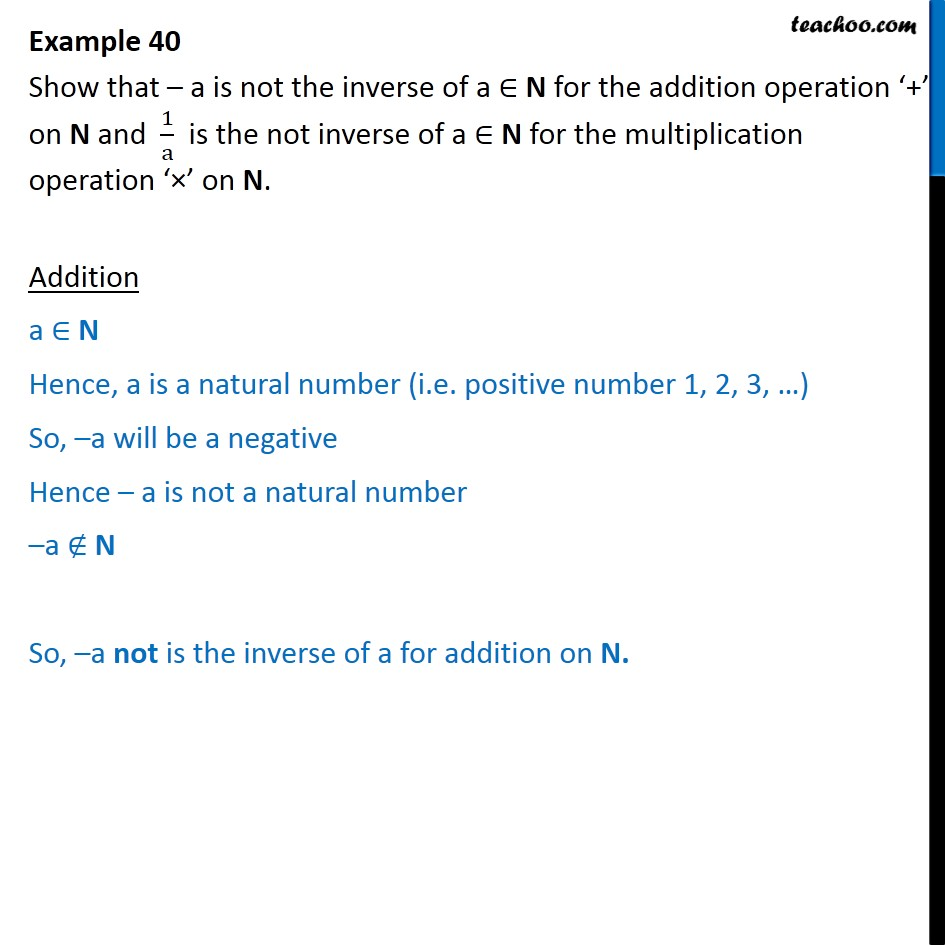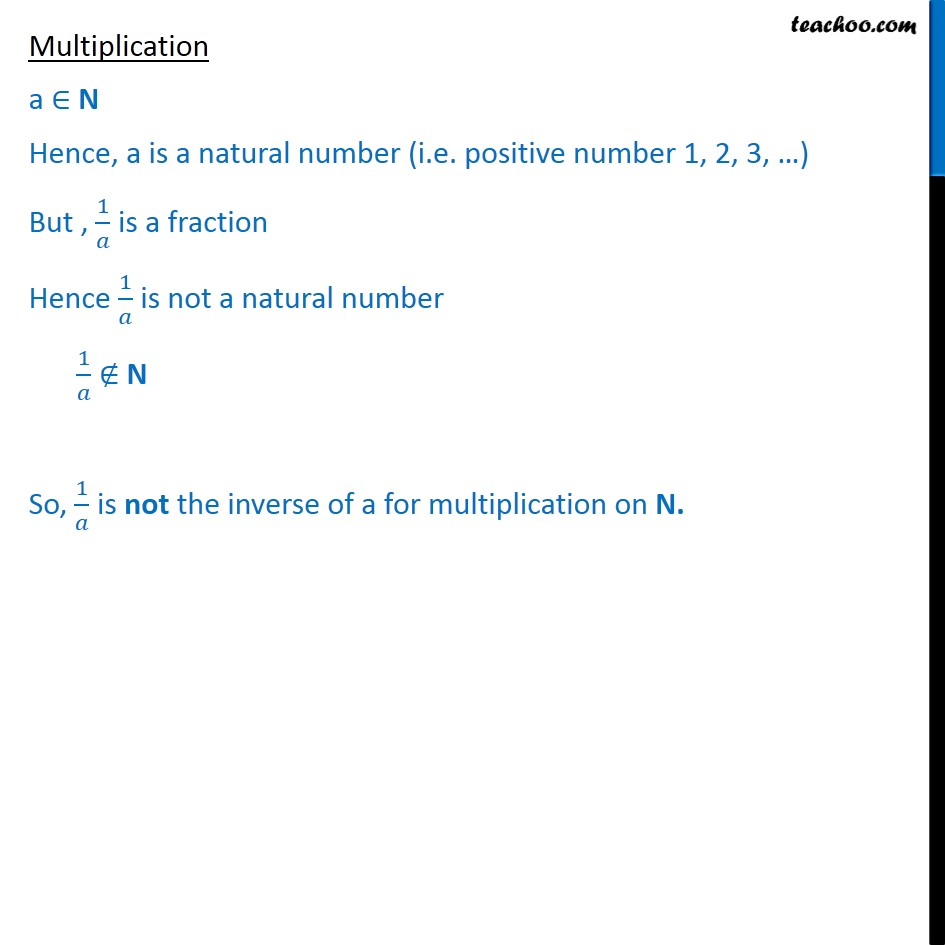Binary operations: Inverse

Chapter 1 Class 12 Relation and Functions
Concept wiseIntroducing your new favourite teacher - Teachoo Black, at only ₹83 per month

### Transcript

Example 40 Show that a is not the inverse of a N for the addition operation + on N and 1 a is the not inverse of a N for the multiplication operation on N. Addition a N Hence, a is a natural number (i.e. positive number 1, 2, 3, ) So, a will be a negative Hence a is not a natural number a N So, a not is the inverse of a for addition on N. Multiplication a N Hence, a is a natural number (i.e. positive number 1, 2, 3, ) But , 1 is a fraction Hence 1 is not a natural number 1 N So, 1 is not the inverse of a for multiplication on N.